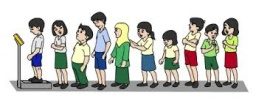# Calculate 6430

There are 600 pupils at the school, of which 350 are boys.

Calculate the ratio

(a) the number of boys to the number of girls
b) the number of girls to the number of pupils at the school.

a =  7:5
b =  5:12

### Step-by-step explanation:Did you find an error or inaccuracy? Feel free to write us. Thank you!

Tips for related online calculators
Need help calculating sum, simplifying, or multiplying fractions? Try our fraction calculator.
Check out our ratio calculator.